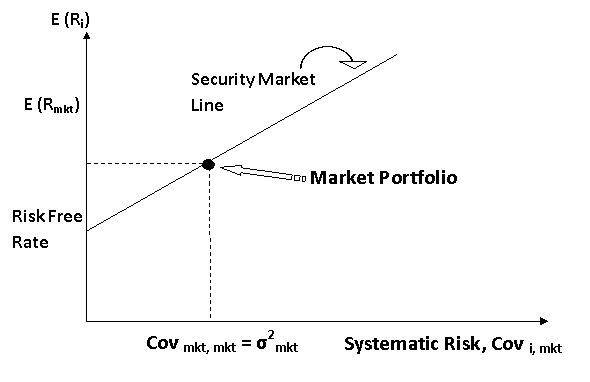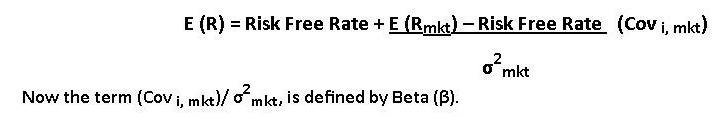# Finance Assignment Help With Capital Asset Pricing Model And Beta

## Capital Asset Pricing Model and Beta

As we have already established that Systematic Risk for an asset is the only relevant risk as it is only accounted while risk and reward are compared. Thus we can plot a graph assuming that the asset i, where the covariance between the assets return and the return on market is Cov i, mkt. Thus this will become our measure of systematic risk as it is d only relevant risk. Plotting it on a graph showing relationship between risk and return we have the Securities Market Line.###The equation of the SML will be,This is the most common way of describing the SML, and this relation between Beta (systematic risk) and expected return is known is the Capital Asset Pricing Model (CAPM)According to CAPM all the securities weather diversified or not will plot on the SML in equilibrium. The CAPM is one of the most fundamental concepts in investment theory. The CAPM is an equilibrium model that predicts the expected return on a stock, given the expected return on the market, the beta coefficient and the risk free rate.

### Following are some of the topics in Risk And Return in which we provide help:

Corporate Finance Homework Help | Finance Assignment Help | Finance Assignment Help | Finance Homework Help | Finance Online Help | Finance Problems Help | Finance Tutor | Help With Finance Homework | Online Tutoring